# imseggeodesic

Segment image into two or three regions using geodesic distance-based color segmentation

## Syntax

``L = imseggeodesic(RGB,BW1,BW2)``
``L = imseggeodesic(RGB,BW1,BW2,BW3)``
``````[L,P] = imseggeodesic(___)``````
``````[L,P] = imseggeodesic(___,Name,Value)``````

## Description

example

````L = imseggeodesic(RGB,BW1,BW2)` segments the color image `RGB`, returning a segmented binary image with labels `L`. `BW1` and `BW2` are binary images that specify the location of the initial seed regions, called scribbles, for the two regions (foreground and background).`imseggeodesic` uses the scribbles specified in `BW1` and `BW2` as representative samples for computing the statistics for their respective regions, which it then uses in segmentation. The scribbles specified by `BW1` and `BW2` (regions that are logical true) should not overlap. The underlying algorithm uses the statistics estimated over the regions marked by the scribbles for segmentation. The greater the number of pixels marked by scribbles, the more accurate the estimation of the region statistics, which typically leads to more accurate segmentation. Therefore, it is a good practice to provide as many scribbles as possible. Typically, provide at least a few hundred pixels as scribbles for each region.```

example

````L = imseggeodesic(RGB,BW1,BW2,BW3)` segments the color image `RGB`, returning a segmented image with three segments (trinary segmentation) with the region labels specified by label matrix `L`. `BW1`, `BW2`, and `BW3` are binary images that specify the location of the initial seed regions or scribbles for the three regions. The scribbles specified by `BW1`, `BW2`, and `BW3` (regions that are logical true) should not overlap.```

example

``````[L,P] = imseggeodesic(___)``` also returns the probability for each pixel belonging to each of the labels in matrix `P`.```

example

``````[L,P] = imseggeodesic(___,Name,Value)``` uses name-value pairs to control aspects of segmentation.```

## Examples

collapse all

```RGB = imread('yellowlily.jpg'); imshow(RGB)```The goal is to segment the petals of the flower. Specify the initial seed region as a rectangular ROI by using the `drawrectangle` function. Display the ROI in red. The 'Position' name-value pair argument specifies the upper left coordinate, width, and height of the ROI as the 4-element vector `[xmin, ymin, width, height]`. If you want to draw the rectangle interactively, then omit the 'Position' name-value pair argument.

`roiObject = drawrectangle(gca,'Position',[350 700 375 120],'Color','r');`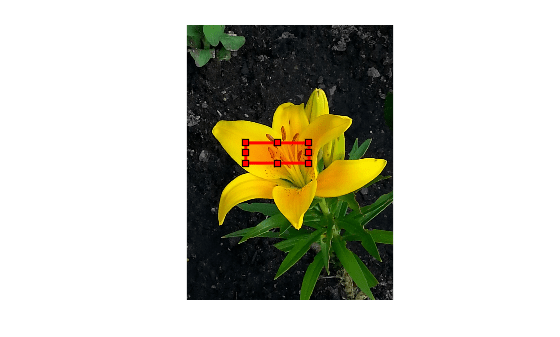Specify the initial seed regions for the background as a rectangular ROI. Display the ROI in blue.

`roiBackground = drawrectangle(gca,'Position',[90 1230 910 190],'Color','b');`Create a mask for each ROI in which the ROI is `true` and other pixels are `false`.

```maskObject = createMask(roiObject); maskBackground = createMask(roiBackground);```

Segment the image.

`[L,P] = imseggeodesic(RGB,maskObject,maskBackground);`

Display the segmented labels.

```imshow(label2rgb(L)) title('Segmented Labels')```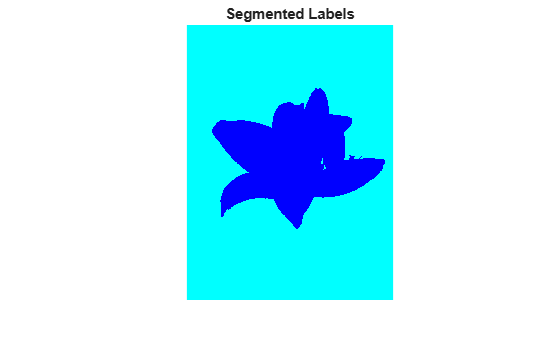Display the segmented labels over the original image.

```imshow(labeloverlay(RGB,L)) title('Labels Overlaid on Original Image')```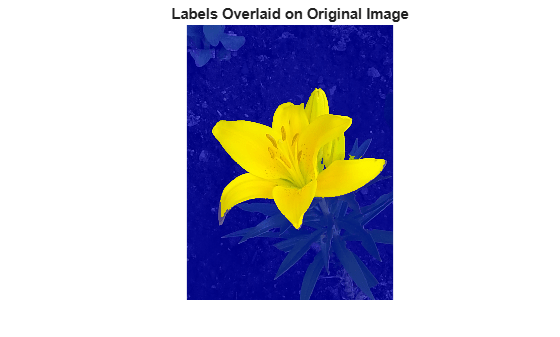```RGB = imread('yellowlily.jpg'); imshow(RGB)```The first region consists of the yellow flower petals. Specify the initial seed region as a rectangular ROI by using the `drawrectangle` function. Draw the ROI in yellow. The 'Position' name-value pair argument specifies the upper left coordinate, width, and height of the ROI as the 4-element vector `[xmin, ymin, width, height]`. If you want to draw the rectangle interactively, then omit the 'Position' name-value pair argument.

`r1 = drawrectangle(gca,'Position',[350 700 425 120],'Color','y');`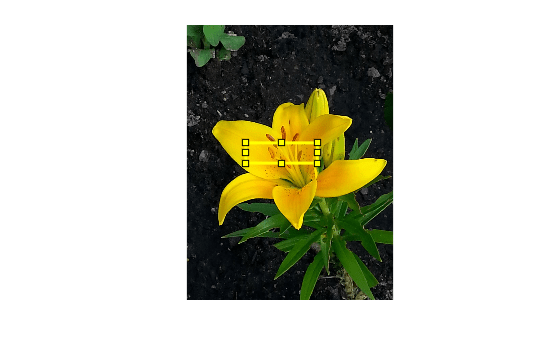The second region consists of the green leaves. Specify the seed region as a rectangular ROI and draw the ROI in red.

`r2 = drawrectangle(gca,'Position',[800 1124 120 230],'Color','r');`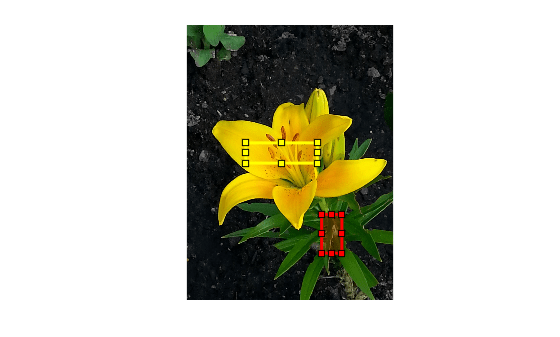The third region is background, which is the dirt in this image. Specify the seed region as a rectangular ROI and draw the ROI in blue.

`r3 = drawrectangle(gca,'Position',[1010 290 180 240],'Color','b');`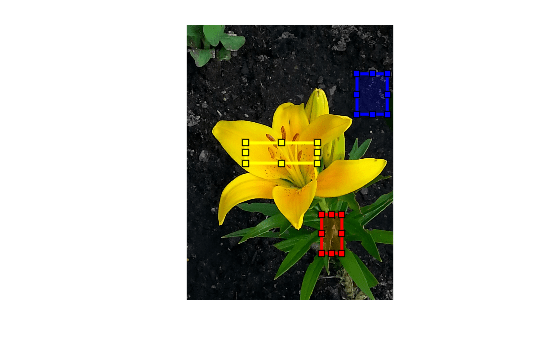Create a mask for each ROI in which the ROI is `true` and other pixels are `false`.

```mask1 = createMask(r1); mask2 = createMask(r2); mask3 = createMask(r3);```

Segment the image.

`[L,P] = imseggeodesic(RGB,mask1,mask2,mask3,'AdaptiveChannelWeighting',true);`

Display the segmented labels over the original image.

```imshow(labeloverlay(RGB,L)) title('Segmented Image with Three Regions')```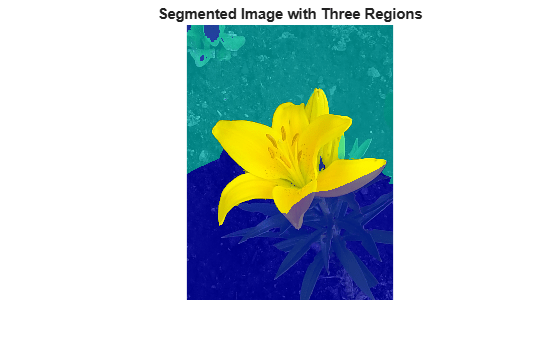The lower right corner of the image is misclassified as region 2 (leaves). Add another background ROI.

`r4 = drawrectangle(gca,'Position',[20 1320 480 200],'Color','b');`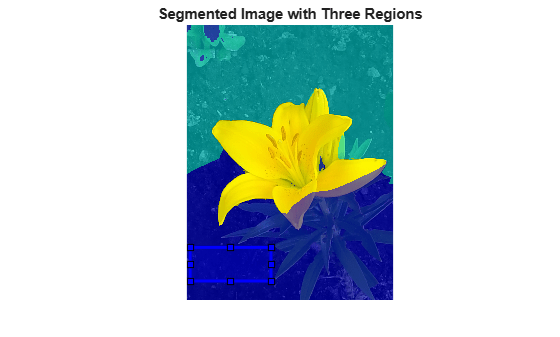```mask4 = createMask(r4); maskBackground = mask3 + mask4;```

Segment the image then display the segmented labels over the original image.

```[L,P] = imseggeodesic(RGB,mask1,mask2,maskBackground,'AdaptiveChannelWeighting',true); imshow(labeloverlay(RGB,L)) title('Refined Segmented Image with Three Regions')```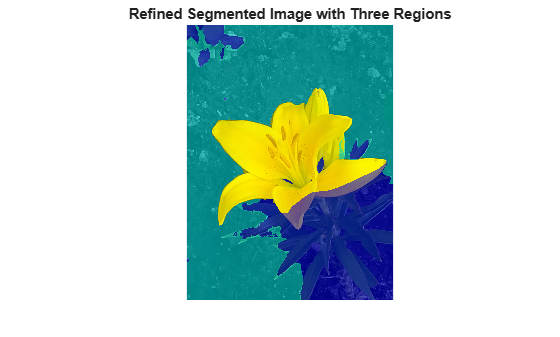Display the probability that each pixel is belongs to each label.

```montage(P,'Size',[1 3]) title('Probability That Each Pixel Belongs to Each Label')```## Input Arguments

collapse all

Image to be segmented, specified as an RGB image. `imseggeodesic` converts the input RGB image to the YCbCr color space before performing the segmentation.

Example: `RGB = imread('peppers.png');`

Data Types: `double` | `uint8` | `uint16`

Scribble image for the first region, specified as a logical matrix. `BW1` must have the same number of rows and columns as the input image `RGB`. To create the scribbles interactively, first draw an ROI using functions such as `drawcircle`, `drawfreehand`, `drawpolygon`, or `drawrectangle`. Then, create a mask from the ROI using `createMask`.

Data Types: `logical`

Scribble image for the second region, specified as a logical matrix. `BW2` must have the same number of rows and columns as the input image `RGB`. To create the scribbles interactively, first draw an ROI using functions such as `drawcircle`, `drawfreehand`, `drawpolygon`, or `drawrectangle`. Then, create a mask from the ROI using `createMask`.

Data Types: `logical`

Scribble image for the third region, specified as a logical matrix. `BW3` must have the same number of rows and columns as the input image `RGB`. To create the scribbles interactively, first draw an ROI using functions such as `drawcircle`, `drawfreehand`, `drawpolygon`, or `drawrectangle`. Then, create a mask from the ROI using `createMask`.

Data Types: `logical`

### Name-Value Arguments

Specify optional pairs of arguments as `Name1=Value1,...,NameN=ValueN`, where `Name` is the argument name and `Value` is the corresponding value. Name-value arguments must appear after other arguments, but the order of the pairs does not matter.

Before R2021a, use commas to separate each name and value, and enclose `Name` in quotes.

Example: ```[L,P] = imseggeodesic(RGB,BW1,BW2,BW3,'AdaptiveChannelWeighting',true);```

Use adaptive channel weighting, specified as a logical scalar. When `true`, `imseggeodesic` weights the channels proportional to the amount of discriminatory information they have that is useful for segmentation (based on the scribbles provided as input). When `false` (the default), `imseggeodesic` weights all the channels equally.

Data Types: `logical`

## Output Arguments

collapse all

Label matrix, returned as a matrix of nonnegative integers. Pixels labeled `0` are the background and pixels labeled `1` identify a segmented region. Pixels labeled `2` identify another segmented region in trinary segmentation.

Data Types: `double`

Probability a pixel belongs to a labeled region, specified as an M-by-N-by-2 matrix for binary segmentation or an M-by-N-by-3 matrix for trinary segmentation. M and N are the number of rows and columns in the input image. `P(i,j,k)` specifies the probability of pixel at location `(i,j)` belonging to label `k`.

Data Types: `double`

## Tips

• The scribbles for the two (or three) regions should not overlap each other. Each scribble matrix (`BW1`, `BW2`, and `BW3`) should be nonempty, that is, there should be at least one pixel (although the more the better) marked as logical `true` in each of the scribbles.

## Algorithms

`imseggeodesic` uses a geodesic distance-based color segmentation algorithm (similar to ).

 A. Protiere and G. Sapiro, Interactive Image Segmentation via Adaptive Weighted Distances, IEEE Transactions on Image Processing. Volume 16, Issue 4, 2007.